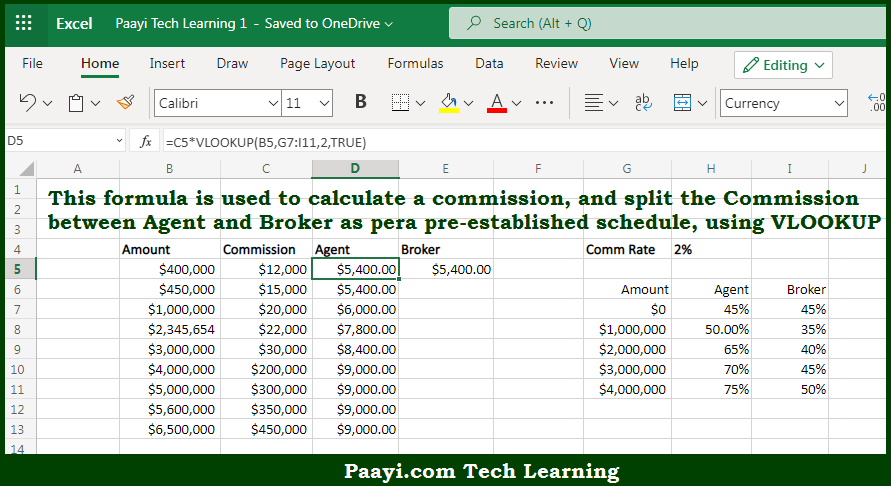# Learn Commission Split Formula Example in Microsoft Excel

Written by | 0 Comments | 337 Views

In this article, you will learn how to evaluate things based on the LOOKUP function in Microsoft Excel using a single/combination(s) of functions. You will also know the Commission Split Formula Example and see the generic formula.

Learn Commission Split Formula Example in Microsoft Excel

The main purpose of this formula is to calculate a commission and split the Commission between Agent and Broker as per the pre-established norms. Here we will learn the commission split formula example in the given data range in the workbook in Microsoft Excel. That implies, with the help of a formula based on the VLOOKUP function you can able to calculate a commission, and split the Commission between Agent and Broker according to a pre-established schedule. So, with the help of this formula, you can able to get the commission split formula example in the given data range in the workbook in Microsoft Excel.

General Formula to Commission Split Formula Example

=commission*VLOOKUP(amount,split_table,column,TRUE)

The Explanation to Commission Split Formula ExampleSo we know that with the help of the given formula above you can able to calculate a commission, and split the Commission between Agent and Broker as per the pre-established norms. Here we will learn the commission split formula example in the given data range in the workbook in Microsoft Excel. As we know that while doing an approximate match, the VLOOKUP function assumes the table is sorted in ascending order. In case the VLOOKUP function gets an exact match, it will return a value from the matching row. If it is a value greater than the lookup value, it will return a value from the previous row. So now you have learned how to perform a commission split formula example in the given data range in the workbook in Microsoft Excel.